# An Elementary Course on Turbocompressors

## 3.1 Actual Processes x Ideal Processes

Imagine you are standing in front of a turbocompressor which is operating in steady state. And for whatever reason, you want to represent the compression process developed by the machine using for this a thermodynamic P-T diagram. Then you should produce something like what we see in the figure 3.1. There we see the points depicting the initial and final states of the compression, respectively 1 and 2. Both can be identified by the readings of pressure and temperature certainly existing as part of the compressor instrumentation.

Much more common when representing thermodynamic processes is to link the two states, at least to indicate the beginning and the end of the process. In this case we firmly advise you to use a dashed sinuous line for this purpose, like can be seen in figure 3.2. This is important to emphasize that the intermediate states of the process have no thermodynamic meaning, as they are non-equilibrium conditions

Whenever you see somebody conjecturing about the path described by a process displayed in a diagram of thermodynamic properties, put in your mind that you are watching a sort of simplified analysis which practical validity can eventually be questioned.

The mapping of continuous lines in thermodynamic diagrams must be conventionally restricted to ideal processes representation, which are mainly used for modelling or benchmarking actual processes.

For a given set of a compressor service conditions (suction pressure, suction temperature, discharge pressure and gas composition), there is an infinite number of possible real processes to be considered, as pictured in the figure 3.3, each one characterized by a different ending state (2). According to Thermodynamics teachings, two effects determine the placement of the ending state of a compression process: thermal exchanges and energy degradation.

As we are talking about turbocompressors, we won’t be concerned about thermal transfer. Conversely, referring to the performance of this kind of machine, energy degradation is a very meaningful factor. Probably you may have heard about energy degradation, but in case you haven’t, pay attention to the next section.

## 3.2 Compression Energy and Energy Degradation

As stated in a previous lesson, the compression implemented in a turbocompressor involves two simultaneous actions: at the same time the gas is pressurized by the external compressive forces, it is moved from the inlet to the outlet of the machine. Both effects demand energy. The work exerted by the compressive forces is absorbed as internal energy by the gas. The work employed in displacing the gas is needed to overcome the resistance imposed by the pressure force field. (Variations of kinetic energy and gravitational energy should yet be mentioned, but are normally residuals, therefore discardable).

The work done (w) related to the compression service, or actual amount of energy incorporated to the gas can be written on massic basis as follows: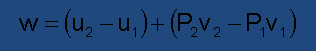(3.01)

Or, according the specific enthalpy (h) definition (h=u+Pv):(3.02)

Eventualy it can be interesting to consider a total work done (wc) representing the total amount of specific work transferred to the compressor. A small fraction of this energy (wf) is dissipated as mechanical friction or used for driving auxiliary equipments.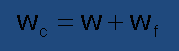(3.03)

The energy dissipated during a turbocompressor operation is normally close to 2% of the total work done or slightly higher when working in reduced loads.

It would be very comfortable to say that Δu in equation (3.01) corresponds to the work done by the compressive forces, while Δ(Pv) corresponds to the work done in gas transportation across the pressure field. However, it is known that the movement through force fields, like the flow of fluids, unavoidably generate dissipative effects which cause the convertion of transport energy into internal energy.

Fluid friction and turbulence are the factors responsible for energy degradation in fluid systems. Because of these factors, the amount of energy needed to move the gas between two points across the pressure forces field is always higher than the corresponding potential energy difference Δ(Pv). The apparent losses turn up as internal energy.

It’s much like the phenomenon observed when a mass block is pushed upwards along an inclined plane. In reason of the friction between the parts, the work needed to move the block is higher than the corresponding potential energy variation (in this case we are referring to gravitational potential energy).

Let’s summarize the question as follows:

1. In order to take the gas from a given initial state to a defined discharge pressure you must employ 20 to 30% more energy than it would be needed without energy degradation.

2. This additional amount of energy is embodied as internal energy. Internal energy is closely related to temperature and therefore the discharged gas is hotter than it would be without energy degradation.

## 3.3 The Main Types of Compression Problems

The principles of thermodynamic analysis are used mainly for the soluion of two types of compression problems:

• The Test Problem
• The Design Problem

They employ the same thermodynamic concepts, but are fundamentally different in their purposes.

In “test type” problems, the compressor is working in front of you, in such a way that it‘s possible to measure, directly or indirectly, the operational parameters of the system. These measurements will serve as basis for the problem solution.

In “design type ” problems the analysis is focused in a virtual operating condition, which is not happening yet, except in someone‘s imagination.

Test problems are normally used for the performance assessment of a compressor or compression plant. On the other hand, design problems arise during the operational planning of compression systems or studies related to specification of machines for acquisition or revamping.

Now considering the application of the first law of Thermodynamics to turbocompressors operation analysis, as before mentioned: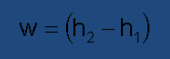(3.04)

This formula can be straightlforwardly used in test problems, because the final thermodynamic state of the compression process is always known. But the same is not true in design problems, when the process final state has to be drawn from thermodynamic calculations.

Objectively, imagine that you are asked for solving the following very common question: Given the service conditions of a compression system, how to estimate the specific energy (head) consumption of a compressor? This is, of course, a matter of interest, in order to anticipate system power needs, among other reasons.

The answer is: it‘s not possible, except you are given some insight into how to found the final state of the compression process that is being devised. Actually, the solution of this kind of problem is conducted through the concept of Efficiency. That’s because, as we saw earlier, the amount of energy degradation along the process rules the placement of its ending state.

## 3.4 Ideal Work and Efficiency

Thermodynamics provides us the means to calculate the ideal specific work involved in simultaneous compression and transport of a unity mass of gas through a control volume (in this case, the compressor), since two simplifying hypothesis are presumed:

1. The “gas path” developed along the control volume can be described by a known continuous function of gas pressure and specific volume.

2. The gas flows free of any dissipative effects like friction and turbulence, which could cause transport energy degradation.

In these conditions, the ideal work (wth) can be found by the equation:(3.05)

Even though the two hypothesis before mentioned are far from to be achievable in real life, they produce a compression model that is very useful as a benchmark for efficiency. We call it the ideal compression process.

We won’t show here any proof of this statement, inasmuch the matter is more suitable to Thermodynamics books. Anyway, we want to point out some important details about the result:

1. This expression is more often encountered with a “minus” signal placed before it. The reason is that work exerted from outside to inside a system is conventionally considered as negative for purpose of using the first law of Thermodynamics, and the integral of v.dp would be always positive when applied to compression processes (because pressure is intrinsically decrescent). We see no need for adopting the same here.

2. The above expression is not a simple integral, but rather an integral defined over a line. In other words, we must replace the specific volume by its corresponding function of pressure, thus turning the integral into a simple type one. This explains why the knowledge of a describing P-v function related to the process is absolutely necessary.

3. It‘s also important to notice that the ideal work calculated by the above equation is proportional to the area between the curve and the vertical axis of a P-v diagram, as we see in figure number five.

Once we have settled on the ideal work concept, the thermodynamic efficiency of an actual compression process is naturally derived: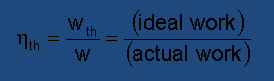(3.06)

provided both, the ideal and the actual processes are developed under the same service conditions (suction pressure, suction temperature, discharge pressure and gas composition).

The ideal compression process is not unique. You can build as many of them as you want, regarding the same actual process, insofar the definition of service conditions opens up this possibility. Notwithstanding, in practice, only two types of ideal processes are employed:

• The ideal polytropic process

Because turbocompressors are, in general, non-cooled machines, the most obvious choice is to consider an ideal adiabatic process for this purpose. We have already shown that this kind of process, when developed by perfect gases, is acceptably represented by the equation: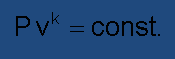(3.07)

where the exponent k is assumed to be a constant. In this case the integral of v.dp results:(3.08)

Or, introducing the perfect gas relationships Pv=RT and R=Ru/MW: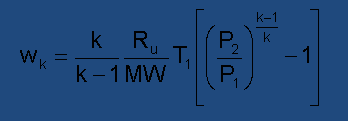(3.09)

Some very useful conclusions can be drawn from this equation:

1. Specific work is straightly dependent on the initial temperature of the compression and inversely dependent on the molecular weight of the gas.

2. Specific work varies with pressure ratio instead of pressure difference, as occurs in liquid pumping.

3. The amount of work required for taking the gas from initial pressure and temperature to a given final pressure increases with the adiabatic exponent k.

4. The adiabatic theoretical work is exclusively dependent on service conditions, and therefore can be anticipated as soon as the service parameters are known.

Applying our definition of thermodynamic efficiency to the specific situation in which the ideal process is adiabatic, we get: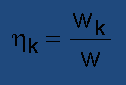(3.10)

This parameter is called adiabatic efficiency, tagged by the subscript ‘k’.

Despite of some conveniences, the adiabatic model for compression processes is hardly ever used in applications of turbocompressors. In this case, the polytropic model, to be presented in the next section, is preferable.

## 3.6 The Polytropic Model

Polytropic processes were initially conceived to represent certain type of perfect gases behavior. However, in many cases similar principles can be adopted in the development of real gas compression studies, without causing fatal errors. In the simplest way, a polytropic ideal process can be defined as the one that follows the equation: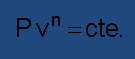(3.11)

Through any pair of ponts selected in a P-v thermodynamic diagram is possible to pass one unique polytropic curve. In order to consider the polytropic process as an ideality model for compression processes, we choose the exponent “n” in such way the curve passes by either the initial and the final points of the actual process, as shown in the figure 3.6. There we see an actual process, depicted as a curvaceous dashed line and the correspondent polytropic ideal process, as a continuous line.

Thus, unlike the adiabatic exponent k, which is a thermodynamic property, the polytropic exponent is simply a mathematical figure. It can be found from the process equation:(3.12)

Or, if you prefer, using the polytropic exponent equation in terms of P and T: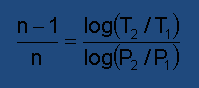(3.13)

You should have already noted that the polytropic equation is mathematically identical to the adiabatic equation, the two being distinguished by the exponent value.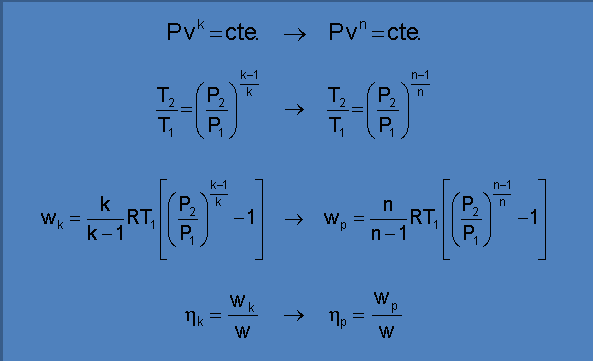In the figure 3.7, for the sake of comparison, the adiabatic curve and the polytropic curve related to a same actual non-cooled process were outlined in a P-v diagram.

The two processes end up in different states. The polytropic final state (2) is on the right of the adiabatic final state (2k) because of the non-idealities. As the actual process is also adiabatic (although non-isentropic), the only reason for the displacement of 2 with respect to 2k is the occurrence of energy degradation.

Therefore, the “distance” between points 2 and 2k may be expected to provide us an accurate inference of the efficiency. In fact, starting from the definition of polytropic efficiency of an adiabatic process developed by a perfect gas and making the proper arrangements: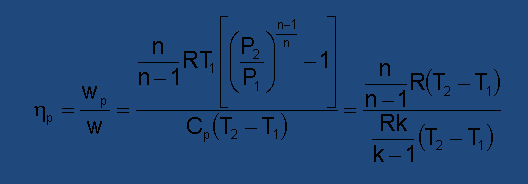(3.14)

we get: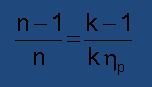(3.15)

This interesting equation highlights the merit of the polytropic efficiency as an exact measurement of the non-ideality degree of adiabatic compression processes.

On the other side, if the actual process was cooled, the rejected heat would mask the energy degradation effects on the compressor efficiency. That is why the use of polytropic efficiency is practically restricted to adiabatic (non-cooled) actual processes, like those developed by turbocompressors.

From now on, our attentions will be focused on methodologies of compression analysis inspired in the polytropic model because they are widely prevalent in current practice.

## 3.7 Analytical and Numerical Approaches

If you have already searched for information about thermodynamic compression calculations on the many existing books that address this subject, probably you have been faced to different approaches to solve the same problems. Didatically it’s interesting to consider two groups of procedures:

- Analytical Approach: In this case the solutions have to be limited to the employment of algebric equations, although eventually requiring iterative procedures. The main drawback of these methodologies is the need of assuming several simplifications in order to make feasible the calculations. Conversely, analytical approaches allow us to understand the way each parameter included affects the results of the problem, and the respective significance.

- Numerical Approach: The solution of the problem is structured to make use of computational processing. The main advantages are the fastness in obtaining the results, besides accuracy and reliability gains. However, the role played by each parameter included in the problem cannot be unveiled without an awkward sensitivity analysis.

It is surprising that most of experimented compressors users still today adopt very simplified approaches for thermodynamic analysis of gases compression. Of course, we must also recognize that these rough procedures can give satisfactory answers to some questions that arise during the daily work routine. However, with the argument of convenience, people end up using these shortcuts for every purpose, frequently causing serious mistakes.

Have in mind that analytical solutions are only possible under unavoidable simplifications, and this means that adaptations have to be made on the gas model. The bad consequences of this are two:

- The inaccuracies may be excessive as the gases move away from the perfect gas condition. Troubles are particularly expectable in high pressure (high density) applications, because the excessive deviation from ideality exhibited by the gas under these circumstances.

- Different calculation methodologies, even in small details, can be applied to the solution of a same problem. Thus, it is necessary to save and analyze the records of all the procedures used to solve each problem, together with the results obtained.

## 3.8 The Analytical Approach on Polytropic Basis

Problems involving thermodynamic calculations of compression processes can be formulated in some different ways, depending on what parameters are assumed as known data and what parameters are to be calculated. Previously, we have referred to the two more important kind of problems, respectively the test type problem and the design type problem. Now we’re going to show how to proceed with the solutions of these problems, step by step, in cases where the polytropic basis is adopted.

## 3.8.1 The Analytical Solution of the Test Problem

In test problems, the compressor is running under a known set of test conditions (suction pressure, suction temperature, discharge pressure, discharge temperature and gas composition) and the corresponding ideal thermodynamic work and thermodynamic efficiency must be found.

Let’s consider a specific problem to illustrate the solution.

Application Example: Methane is compressed by an industrial turbocompressor. The following pressures and temperatures of suction and discharge were carefully measured in the system: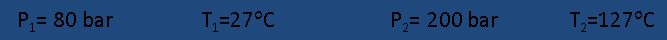The molecular weight of the methane is 16.043 and its specific heat capacity at constant pressure can be found by the equation: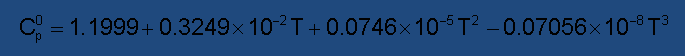(The temperatures must be entered in degrees Kelvin).

Determine the polytropic head and the polytropic efficiency of the process.

Solution:

Step number one: Find the gas constant.Step number two: Find the average adiabatic exponent and the average compressibility factors related to the compression process. This is a simple task, because both the initial state and the final state of the process are known.

For simplicity, assume the perfect gas behavior, and thus both k and Cp become exclusively temperature dependent functions. The k value at suction temperature (k1) and at discharge temperature (k2) can be found as a function of the specific heat capacity at each one of these temperatures, respectively which are easy to calculate.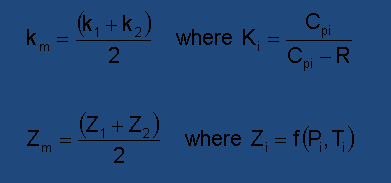Solving, we get:Be aware that the compressibility factor can be calculated by different equations, depending on the assumed P-v-T behavior, so that small differences may result between answers to the same problem but solved by different means.

Step number three: Find the polytropic exponent “n ” using measured pressures and temperatures.In our case: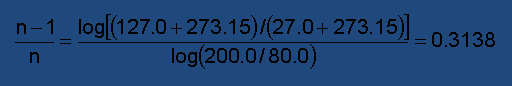Actually we need only the value of (n-1)/n, but if you want to know, n=1.4574

Step number four: Find the ideal polytropic work by the formula: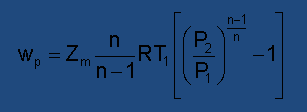Here the average compressibility factor was included as a corrective factor applied to the theoretical expression of the polytropic work. There is a common belief that this procedure leads to a better accuracy when dealing with non-perfect gases. In our experience, this is generally true. But be careful if the compressed gas presents a very large departure from perfect gas condition, because the result obtained can be worsened, instead of being improved.

Proceeding with the calculations we have: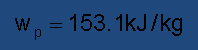Step number five: Find the polytropic efficiency using the relationship between the exponents “n” and “k”: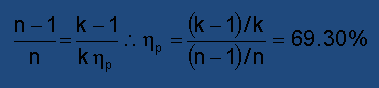That’s all. Very easy, isn’t it? In trivial test problems, all we need to calculate is the ideal polytropic work and the respective polytropic efficiency. The knowledge of these quantities obtained during a performance test will be fundamental for future studies about the compressor and the compression system.

## 3.8.2 The Analytical Solution of the Design Problem

In design problems the input data are the compressor service conditions (suction pressure, suction temperature, discharge pressure and gas composition) together with the process polytropic efficiency, while the actual work and the discharge temperature have to be found.

The design type problem requires trial and error solution, because the final state of the compression is unknown. The appropriate strategy is to make an initial guess for the discharge temperature and to check it after the duly calculations.

In the same way we did in previous section, now we shall exhibit the solution of the design problem by exploring a concrete example.

Application Example:Suppose we have to find out the amount of specific energy (Head) to be dispensed to a carbon dioxide turbocompressor, together with the final temperature expected for the gas, referring to the service described below.Solution:

Step number one: Find the gas constant of the carbon dioxide.Step number two: Make a guess about the value of the compressor discharge temperature. Use pure intuition, perfect gas relationships or whatever you want. We will choose here T2=163,1 °C without any further explanation.

At this point we start the iterative procedure, performing cyclically the three following steps.

Step number three: Calculate the average adiabatic exponent using the discharge temperature just assumed.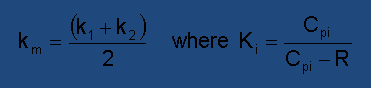Step number four: Calculate the polytropic exponent combining the average adiabatic exponent and the known polytropic efficiency: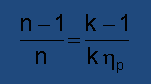Step number five: Calculate the discharge temperature based on the value of (n-1)/n encountered in the previous step.Step number six: Compare the discharge temperature that has just been found to the initial guess about this parameter. If they are sufficiently close to each other, we can stop with the iterations, assuming that the last value of T2 is the actual discharge temperature. Otherwise, we must go back to the third step with this new value.

In our application we needed three cycles of iteration to get a satisfactory convergency. See below an overview of the results of our attempts.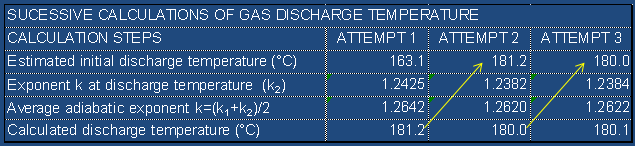The expected discharge gas temperature is 180,1 °C. From now on the calcul is straight, non-iterative.

Step number seven: Calculate the average compressibility factor corresponding to the initial and final states of the compression, using the appropriate resource for the gas being compressed: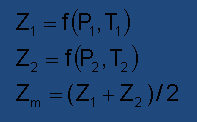Step number eight: Calculate the ideal polytropic work by the formula: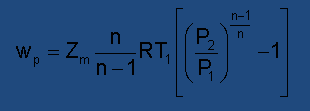Step number nine: Calculate the actual compression work by means of: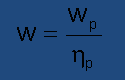Here we have the summary of our last calculations. Numbers in red are the final answers to the problem.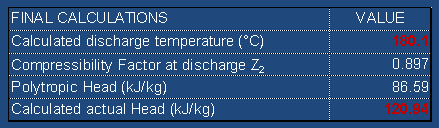## 3.8.3 How Trustable is a Compression Process Calculation

As soon as you finish any kind of compression process calculation, a doubt arises: “How can we know if these numbers are correct?”

- “We can’t”, it’s the proper answer to this question. The reason is very simple: the ideal processes are imaginary figures, they don’t exist physically. The polytropic work and the polytropic efficiency result from rules arbitrarily established by the free conception of the ideality model. Different conceptions, or even different criteria used in calcul approximations can lead to multiple valid solutions for a same problem. We have to stick with one of them.

The contribution of the standards or reference papers to enlighten this complicated subject leaves too much to be desired. The compressor documents almost never state clearly what procedures have been used to generate some thermodynamic data encountered in the supplied data-sheets. References to parameters such as “average adiabatic exponent” or “average compressibility factor” suggests that some kind of approximation have to be performed, but without going into the details on how to determine and how to apply these data. This is not good because it prevents a very important rule from being observed: “The empirical or theoretical parameters involved in compression calculations should be applied in absolute agreement with the way they were generated”.

Never forget that the “design type” and “test type” problems are reciprocal problems. A parameter like polytropic efficiency, for instance, must be manipulated in the design problem accordingly the same criteria adopted in the test that originated it.

Let’s get back to the question of evaluating the result of a polytropic analysis. Although it is not possible to directly check the accuracy of the calculations, there are two ways to test the consistency of the resulting data.

The first one is based on the measurement of the flow energy. The actual work done (ratio between polytropic work and polytropic efficiency) of a turbocompressor must be equal to the gas enthalpy difference, as we have seen previously. Thus, the compliance with this thermodynamic inference can be evaluated as a consistency index: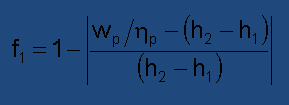(3.16)

The higher this value, the higher the consistency of the solution.

The enthalpy difference depends on the initial and final states of the process, besides the gas composition and specially the P-v-T behavior assumed for the gas. A good practice is to repeat the calculations several times, using distinct equations of state, in order to obtain an average value for this quantity, probably enhancing the accuracy of the procedure.

In due time we will discuss a little about enthalpy calculations, but the more important is to get access to the modern softwares dedicated to fluid properties prediction, which are very helpful to this purpose.

The second method available for checking the consistency of performance analysis is based on the practical determination of the power transferred from the driver to the compressor, and is only applicable to test activities. The incoming power (Wm) divided by the gas mass flow rate (M) and multiplied by the compressor mechanical efficiency (ηmec) gives raise to the actual work of compression (wm), now calculated from the driver’s point of view. This quantity can be compared to the actual work as calculated from the polytropic analysis, producing another type of consistency index: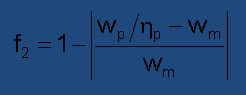(3.17)

Unhappily, the incoming power cannot be easily determined for all species of drivers. Except the eletric motors and the non-condensable steam turbines, the power deliverance of the other drivers is hard to obtain with the requested accuracy. Moreover, the inclusion of the gas flow rate measurement brings an additional uncertainty to this methodology.

Let us see some numbers.

In section 3.8.1 we presented data on a test of a methane compressor and we found out the following test results: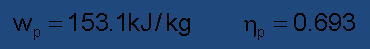Based on published information about methane properties, we know that the entalphy difference between the initial and final states observed in the test is: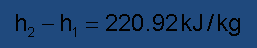Taking into our first consistence formula:This represents 98.5% of precision, not bad regarding the average compressibility factor of the process, 0.9272.

Moving on to the application focused in section 3.8.2 referring to a carbon dioxide compression design, we found a polytropic head of 86.59 kJ/kg. The polytropic efficiency supplied by the problem was 71.6%. Consequently, the calculated actual head was 120.94 kJ/kg. Using a manual of carbon dioxide properties we can get the actual entalphy variation of the gas in this process: 122.57 kJ/kg. Leading into the consistence test formula: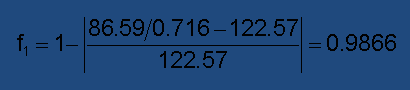This result gives us a good expectance about the accuracy of the performed calculations, despite the relativelly high non-ideality of the compressed gas indicated by an average compressibility factor of 0.886.

We suggest that costumers and manufacturers pay attention to the possibility of using these consistency parameters as an acceptance criteria for compressors performance analysis. Better yet if a clause regarding compliance with this recommendation would be included in the regulatory documents applicable to the practices of thermodynamic analysis of compression processes.

## 3.9. Solution Strategy for Compression Numerical Analysis

In order to perform the numerical analysis of a compression processes, it is first necessary to obtain a suitable computational support. Then you start the computer program, choose the problem type, fill in the blanks with the compression data, press a button to get your answers and that’s it.

What we are going to show here is a brief summary of the strategy that takes place in the backstage of these routines.

The perception that the equation(3.18)

when used for real gases doesn’t provide the same conveniences than those obtained for perfect gases has motivated the search for alternatives since a long time ago.The work of Edmister1 and Schultz2 are considered the most prominent contributions in this sense, having the second gained wider notoriety because the more clear and objective approach given to the subject. Both adopted the redefinition of the polytropic process as being the one carried out between the same initial and final states pertaining to the actual process, along which the ratio(3.19)

remains constant. If we restrict our interest to adiabatic compressors, like turbocompressors, this quantity happens to be a differential expression of the polytropic efficiency:(3.20)

The denominator of the fraction, dh, represents a small increment of enthalpy associated to the polytropic process. Furthermore, it will correspond, after integration, to the enthalpy change of the actual process, because of the coincidence, by definition, of the extreme states of both processes.

Schultz derived the relationships respectively between P and v and between P and T over a path oriented by a constant arbitrarily defined value of the polytropic efficiency and put the results in a very interesting form, shown below: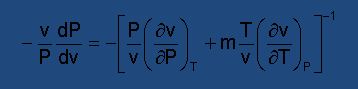(3.21)(3.22)

The convenience of these relationships lies in the fact that, if their right members were constants, the corresponding equations would exactly match the differential forms that give raise to the parameters n and m encountered in perfect gas equivalent formulations. In other words, admitting that the right side members of the above equations were constants represented by n and m, these relationships would be analitically integrable, resulting in: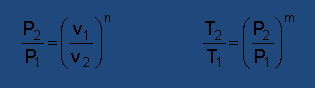(3.23)

However, the parameters n and m are complex functions of several thermodynamic properties, so that no useful algebraic form of solution can be achieved from these equations.

At the time Schultz wrote his paper entitled “The Polytropic Analysis of Centrifugal Compressors”, the available computational resources were very incipient, and he couldn’t outline the better ways to apply his theory. Instead, he suggested analytical forms of solution that ignored the variability of parameters n and m, replacing each one by the respective average between the values at both ends of the process. Even today, some people favour the adoption of the simplification proposed by Schultz, arguing that the inaccuracies associated with the determination of the specific heat at constant pressure and also to partial derivatives involved in the equations may not justify the employment of numerical integration.

Nowadays, with the large disposal of computational means, the Schultz’s approach has been used as basis for numerically solving compression problems.

The general strategy is to divide the ideal polytropic process in many segments that are sufficiently small to allow us to assume the invariability of the parameters involved in the differential equations shown above. Then, the variables Z and Cp, in addition to the partial derivatives related to f(P,v,T)=0 are calculated at the beginning of each segment. And the resulting exponents m and n are used to obtain the final values of pressure and temperature of each segment, as a function of the known initial values of these properties. The final values of pressure and temperature of a segment will be the initial values of the next segment, and so successively, until the end of the process is reached.The outcoming of the last segment will define the ending state of both, the polytropic process and the actual process.

Along the calculation, the small contributions of polytropic work referring to each segment are determined and stored:(3.24)

Here, the indexes 1 and 2 represent the extreme states of a given segment.

Adding these small parts of work, we get the polytropic ideal work related to the whole process: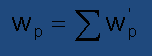(3.25)

The solution strategy for numerical analysis of compression processes that we have just presented is applicable to either, the Test Type and the Design Type problems.

As we have seen, the solution of both types of problems requires the division of the polytropic process in an arbitrary number of segments. The best approach seems to be to do it in such a way that the gas is submitted to the same pressure ratio in all process segments. (So, the pressure ratio of each segment will be equal to the m-th root of the overall pressure ratio, where m is the number of segments).

The only difference is the criterion used to outline the ideal polytropic process correspondent to the actual process being focused, according Schultz’s work.

In Type Test problems, the gas final temperature is known, but the polytropic efficiency is not. The solving procedure is iterative, in which sucessive guesses of the efficiency are formulated, until the final temperature calculated matches to the known value of this property. When it happens, we have got the polytropic work and the polytropic efficiency.

In Design Type problems, the calculation is straight, because the polytropic efficiency is known. After the calculation of the last segment, we have the answers we want, namely work done and final gas temperature.

Bibliographic References:

1. Edmister, W. C.; McGarry, R. J. “Gas Compressor Design”, Chemical Engineering Progress, vol. 45, 1949, pp 421-431.

2. Schultz, J. M. “The Polytropic Analysis of Centrifugal Compressors”, Journal of Engineering for Power (Trans. ASME), Jan 1962, pp 69-82.